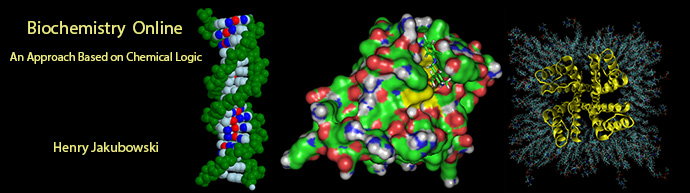# Biochemistry Online: An Approach Based on Chemical Logic# CHAPTER 6 - TRANSPORT AND KINETICS

## B:  Kinetics of Simple and Enzyme-Catalyzed Reactions

BIOCHEMISTRY - DR. JAKUBOWSKI

4/10/16

 Learning Goals/Objectives for Chapter 6B:  After class and this reading, students will be able to write appropriate chemical and differential equations for the rate of disappearance of reactants or appearance of products for 1st order, pseudo first order, second order, reversible first order reactions draw and interpret graphs for integrated rate equations (showing reactant or product concentrations as a function of time) and initial rate equations (showing the initial velocity vo as a function of reactant ; derive kinetic rate constants from data and graphs of integrated rate and initial rate equations; write appropriate chemical, differential equations, and initial rate equations for the rate of disappearance of reactants or appearance of products for simple enzyme catalyzed reaction; differentiate between rapid and steady state assumptions; simplify the initial rate equation containing rate constants for an enzyme catalyzed reactions to one replacing the rate constants with kcat and KM, and give operational and mathematical definitions of those constants;Navigation

Archived version of full Chapter 5B:  Kinetics of Simple and Enzyme-Catalyzed Reactions